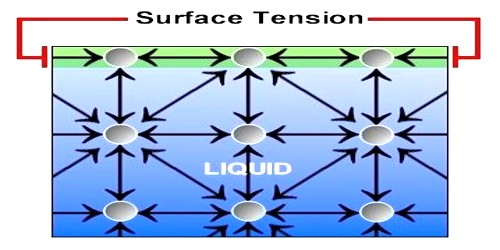# Demonstrate Surface Tension Effect

Surface tension effect can be easily demonstrated by an experiment given below-

When a circular ring of metal wire is dipped inside a soap-liquid and then taken out, there appears a thin film inside the ring. Now, if a small loop made of thread dipping inside the soap solution is placed on the thin film of the ring, it will be seen that the loop keeps itself in random shape (Figure a).Now, if inner portion of the loop is pierced by a pin it will be seen that loop, instead of remaining random, has become circular. (Figure: b).

From the explanation of the above two events it can be said that when there was film inside the loop, then in each point along the tangent of the surface equal and opposite forces were active. As a result in each point these forces balance each other. So the loop is random. But when the loop was pierced, there was no inward force, only the outward force was active, so it experienced outward tension and consequently the loop became stretched and shape was circular. From the above experiment it is evident that one type of tension is active on the free surface of the liquid. This tension is nothing but surface tension. So, surface tension may be defined as follow:-

If a straight line is considered on the surface of a liquid, then on both sides of that line the tangential force per unit length that act on the surface of the liquid is called surface tension.